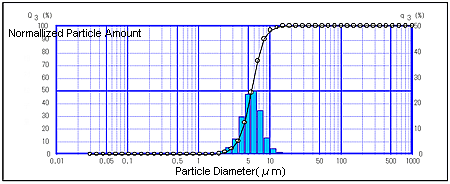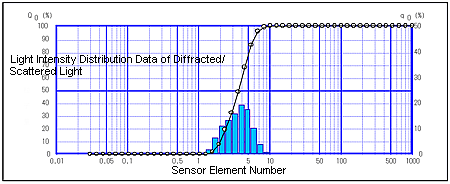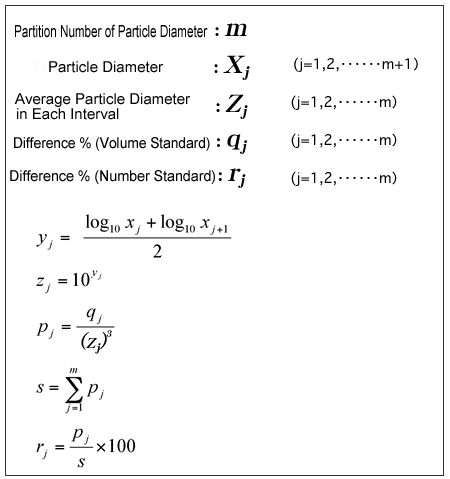# Volume Standard and Number Standard in Particle Size Distribution

Particle size analyzers that adopt the "laser diffraction/scattering method," i.e., laser diffraction particle size analyzers measure the particle size distribution by the volume standard. Note, however, that measurement results can also be output as the particle size distribution according to the number standard through calculation from the volume standard, if necessary.
Generally, most of instruments called "particle size analyzers" apparently measure particle size distribution based on the volume standard. In contrast, on instruments that are referred to by the name "counter," such as a nanoparticle counter, the number standard is used in almost all cases even if a different principle of measurement is used.
The relationship between 1 µm and 10 µm size particles is 1:1000 in the case of the volume standard even though it is 1:1 for the number standard. Inversely, particles exists in a 1000:1 relationship in the case of the number standard even though it is 1:1 for a volume standard. Thus, quite a different impression can be imparted even for the particle size distribution of the same measurement target depending on which of volume standard or number standard is used.
Figs. 1 and 2 show, for example, how the same particle size distribution is expressed depending on which of the volume standard or number standard, respectively, is used. It can be seen that the difference between the two broadens even more in the case of a wide distribution range.

### Fig. 1 Volume Standard### Fig. 2 Number StandardParticle size distribution according to the number standard can be calculated from the particle size distribution according to the volume standard assuming that a particle is spherical. Inversely, the particle size distribution according to the volume standard can be calculated from the particle size distribution according to the number standard. The following shows the formula for converting the different % (volume standard) to difference % (number standard).